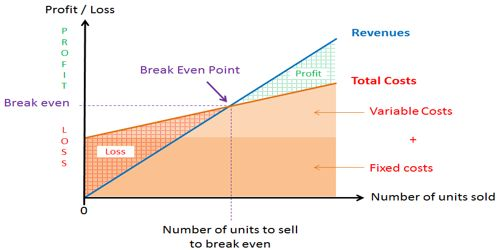Accounting

# An increase in Income Tax Rate affects the Break-Even Point – ExplanationAn increase in Income Tax Rate affects the Break-Even Point

An increase in the income tax rate does not affect the breakeven point. Operating income at the breakeven point is zero, and no income taxes are paid at this point.

The break-even point represents the level of sales revenue that equals the total of the variable and fixed costs for a given volume of output at a particular capacity use rate. Break-even point is that level of operation at which sales revenues for a period are equal to the costs assigned to that period. As a result, there is no net profit or loss. Break-even chart is a graph that shows the relationships among sales, volume, cost and net profit or loss. Contribution margin calculations emphasize the distinction between fixed and variable costs. Hence, the contribution margin is a more useful concept than gross margin in CVP analysis.

For example, one might want to ask the break-even occupancy rate (or vacancy rate) for a hotel or the break-even load rate for an airliner.

An apparent loss may be the result of allocated common costs or of sunk costs that cannot be avoided if the product is dropped. A product should be discontinued only if the contribution margin that will be lost as a result of dropping the product is less than the fixed costs that would be avoided. Even in that situation, the product may be retained if it promotes the sale of other products. There are several reasons why a company’s break-even point will increase. One reason is an increase in the company’s fixed costs, such as rent, depreciation, salaries of managers and executives, etc.